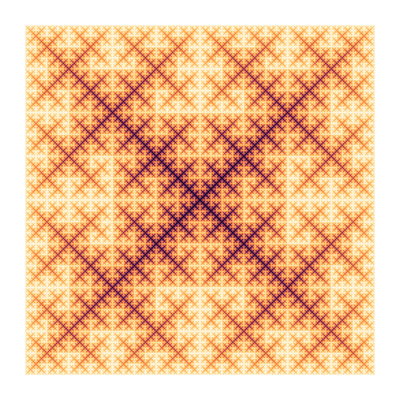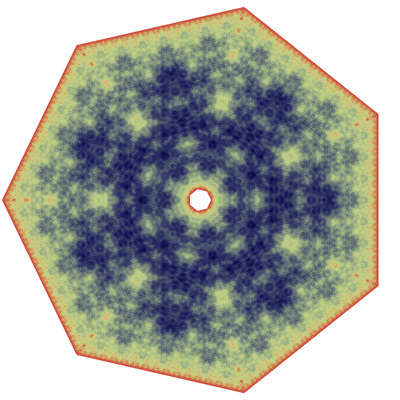# Polygon Chaos Game (IFS)

Written by Paul Bourke
December 2021

The chaos game is well know, although surprising when encountered for the first time. One considers three points on the plane, let's call them seeds. One then starts from any position on the plane and draw a point at that position, then choose a random seed and move from the current point to half way towards that seed and draw the new point. Repeat this a very large number of times. A variation is instead of moving half way towards a randomly chosen seed, choose two different seeds from random and move half way towards their midpoint, drawing the new point and continuing as before. The result is the familiar fractal as shown below, also part of a family of fractals known as iterated function systems (IFS).One can obviously extend this to more than 3 points, here we consider polygonal approximations to a circle. So, for an N vertex approximation the vertices are located at multiples of 360/N around around the circle. In the above N=3, for the following N=4.And the following N=5, a pentagon. As N increases the interior of the polygonal circle increasingly looses any structure and as N increases it just becomes an increasingly diffuse cloud.In order to create structure a further variation can be employed. Instead of choosing two seeds and moving towards their midpoint, one chooses one seed at random and chooses the second seed as being the subsequent seed, or second subsequent seed. The following is the same pentagonal polygon but now choosing the second seed as two after the first randomly chosen seed.The following is a hexagonal polygon and choosing the second seed as the next seed in the hexagon.The following is a septagonal polygon and choosing the second seed as the next seed in the septagon.